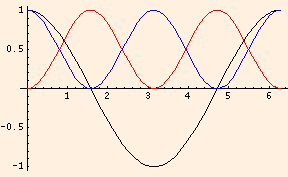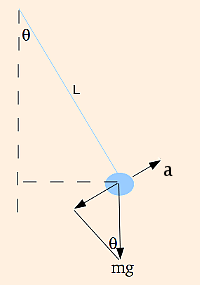### Oscillators

1. The inductance
L = ΦB / I,
is measured in Henrys (1 H ≡ 1 V s / A). It depends only on the geometry of the inductor and the permeability.

• q → I
• τ → L/R

V - q / C - I R = 0 → (q - C V) = - R C dq / dt

V - I R - L dI / dt = 0 → (I - V / R) = - (L / R) dI / dt
2. The energy stored in an inductor is L I2 / 2 (integrate the power over time).

The energy density of a magnetic field is B2 / (2 μ) (this result can be computed using a solenoid:

L I2 / 2 / (area * length) = B * N * I / (2 length)
= B * B * N / (μ N / length * 2 * length)
but it is true in general).
3. The mutual inductance between inductors 1 and 2 is
M12 = Φ1 → 2 / I1,
where Φ1 → 2 is the flux induced in inductor 2 by inductor 1. The induced potential is - M12 dI1/dt.
4. For an LC circuit, Kirchhoff's loop rule gives us (for the discharge cycle):
q / C + L d2q/dt2 = 0.
This has the general solution of a simple harmonic oscillator:
q(t) = A cos (ω t + φ),
where the angular frequency ω = 1 / √ (L C), having units of 1 / s. The argument of the cosine function is called the phase, φ is the phase angle, which is determined by the initial conditions, and A is the amplitude, in this case the maximum charge. The period T = 2 π / ω and the frequency ν = 1 / T, measured in Hertz (Hz).

The simple harmonic oscillator is a conservative system. In this graph, q(t) is in black, q2 / 2 C is in blue and L I2 / 2 is in red:5. For an RLC circuit, the loop rule for the discharge cycle now takes the form:
R dq/dt + q / C + L d2q/dt2 = 0.
If R < √ (4 L / C), it has real solutions
q(t) = A e - R t / (2 L) cos (ω t + φ)
where ω = √ (1 / (L C) - (R / (2 L))2).

If R > √ (4 L / C), ω is imaginary and the circuit is said to be overdamped.

6. The RLC circuit is isomorphic to the damped harmonic oscillator:
m d2x/dt2 + k x + b dx/dt = 0
with the substitutions:

• q → x
• R → b
• L → m
• C → 1 / k
Remember that x represents the displacement from the equilibrium position.
The potential difference supplied by a battery is analogous to an external driving force.
7. The Taylor series for the sine function is
sin θ = Σk = 0 (-1)k θ2k + 1 / (2k + 1)!
so for small angles sin θ ≈ θ.

For small oscillations of the pendulum (with x = L θ):we have

m d2x/dt2 + m g sin θ = 0

d2θ/dt2 + (g / L) θ = 0

which is a simple harmonic oscillator with angular frequency √ (g / L).
8. Even though capacitance is isomorphic to the inverse of the spring constant, the effective spring constant for two springs in series is
keffective = 1 / (1 / k1 + 1 / k2)
and the effective spring constant for springs in parallel is simply the sum of the spring constants. This is due to the fact that in the isomorphism, charge is related to displacement and voltage drop is related to force. For the two capacitors in series, their charges are equal and their voltages add; for the two springs in series, their displacements add while the force (m g) is the same for both.

 (prev) (top) (next)